U.S. Department of Transportation
1200 New Jersey Avenue, SE
Washington, DC 20590
202-366-4000

Federal Highway Administration Research and Technology
Coordinating, Developing, and Delivering Highway Transportation InnovationsThis report is an archived publication and may contain dated technical, contact, and link information
 Federal Highway Administration > Publications > Research Publications > LTPP Publications > 03041 > 05.Cfm > Evaluation and Analysis of LTPP Pavement Layer Thickness Data
 Publication Number: FHWA-RD-03-041

# Evaluation and Analysis of LTPP Pavement Layer Thickness Data

This chapter contains the results of an evaluation of within-section variation in layer thickness values. Characteristics of within-section layer thickness variability are very important inputs in reliability-based pavement engineering applications. This chapter contains the discussion of data sources used for the analysis of within-section variation in layer thickness values, the methodology used to assess characteristics of within-section layer thickness distribution, testing procedures used to evaluate goodness-of-fit between theoretical models and observed layer thickness data, and the results of the within-section layer thickness variability evaluation.

### Data Sources

Data from the elevation measurements were used to evaluate the extent of within-section variation in layer thicknesses. Elevation measurements for each pavement layer were taken along the LTPP section length during the construction phase of the SPS experiments. These data are available in the SPS*_LAYER_THICKNESS tables. Unlike other LTPP layer thickness tables, the data in the SPS*_LAYER_THICKNESS tables are stored not by the layer number but by layer and material type identifiers. Table 30 provides an overview of which identifiers are available in the SPS*_LAYER_THICKNESS tables.

Table 30. Pavement layer and material type identifiers available in the SPS*_LAYER_THICKNESS tables.
Layer and Material Type LTPP Field Name (layer identifier) LTPP Table Name
AC surface courseSURFACE_COURSESPS5_LAYER_THICKNESS,
SPS6_LAYER_THICKNESS
AC binder courseBINDER_COURSESPS5_LAYER_THICKNESS,
SPS6_LAYER_THICKNESS
AC surface and binder courseSURFACE_AND_BINDERSPS1_LAYER_THICKNESS
ASPH_SURFACE_AND_BINDERSPS8_LAYER_THICKNESS
AC surface friction courseSURFACE_FRICTIONSPS1_LAYER_THICKNESS,
SPS5_LAYER_THICKNESS,
SPS6_LAYER_THICKNESS,
SPS8_LAYER_THICKNESS
SPS2_LAYER_THICKNESS,
SPS8_LAYER_THICKNESS
DGATBDENSE_GRD_ASPH_TREAT_BASESPS1_LAYER_THICKNESS
PATBPERM_ASPH_TREAT_BASESPS1_LAYER_THICKNESS,
SPS2_LAYER_THICKNESS
LC baseLEAN_CONCRETESPS2_LAYER_THICKNESS
PCC surface layerPCC_SURFACESPS2_LAYER_THICKNESS
PORT_CEMENT_CONCRETE_SURFACESPS8_LAYER_THICKNESS
PCC overlay layerSURFACE_COURSESPS7_LAYER_THICKNESS
Rut level-up layerRUT_LEVEL_UPSPS5_LAYER_THICKNESS,
SPS6_LAYER_THICKNESS
Mill replacement layerMILL_REPLACESPS5_LAYER_THICKNESS,
SPS6_LAYER_THICKNESS

### SPS Layer Thickness Characteristics

Design Thickness

For a particular SPS experiment, several design thickness values were used as a target design layer thickness. For a given SPS section, only one design thickness value was used along the section length. The design thicknesses for different layers were reviewed for each SPS experiment. Table 31 provides an overview of the material and layer types used in different SPS experiments, the design thicknesses, and the number of layers with the along-the-section thickness measurements available in the LTPP database, Level E version released on June 29, 2001.

Descriptive Layer Thickness Statistics

Using layer thickness measurements along the section, an exploratory data analysis was conducted, and descriptive statistical measures such as mean, standard deviation, kurtosis, skewness, and number of thickness measurements per layer were computed for each structural layer (surface and base courses) that had layer thickness information available in the SPS*_LAYER_THICKNESS tables. These descriptive statistics were then used to evaluate characteristics of layer thickness distribution along the LTPP section.

The following description of the statistical variables provides background information to facilitate the understanding of the procedures used to evaluate within-project layer thickness variability.

The mean is a property of the distribution that describes the location of the distribution. The mean layer thickness is computed as the average of the individual thicknesses obtained from elevation measurements taken along the LTPP section.

The standard deviation is a property of the distribution that describes the spread of the distribution. The standard deviation is based on the second moment of the measurement distribution.

The skewness is a property of the distribution that is used to evaluate how skew the distribution is. The skewness is 0 for a symmetric distribution, positive if the distribution has a long tail to the right, and negative if the distribution has a long tail to the left. The skewness is based on the third moment of the measurement distribution.

The kurtosis is another property of the distribution that provides a mean to evaluate how heavy (or light) the tails of the distribution are. For a normal distribution, the kurtosis is 0. For a distribution with long or fat tails, the kurtosis is positive. For a distribution with short or slim tails, relative to a normal distribution, the kurtosis is negative (but always > -3). The adjusted fourth moment of the measurement distribution is one way to measure the kurtosis of the distribution.

Table 31. Design thicknesses for different SPS experiments sorted by layer and material type.
Layer and Material Type Experiment Type Design Layer Thickness, mm (in) Total Number of Layers used in the Analysis
AC surface course SPS-5 0 93
51 (2)
127 (5)
SPS-6 0 40
102 (4)
203 (8)
AC surface and binder course SPS-1 102 (4) 167
178 (7)
SPS-8 102 (4) 24
178 (7)
AC binder course SPS-5 Varies 33
SPS-6 Varies 17
DGAB SPS-1 102 (4) 97
2023 (8)
305 (12)
SPS-2 102 (4) 85
152 (6)
SPS-8 152 (6) 38
203 (8)
305 (12)
DGATB SPS-1 102 (4) 97
203 (8)
305 (12)
PATB SPS-1 102 (4) 83
SPS-2 102 (4) 47
LC base SPS-2 152 (6) 48
PCC surface layer SPS-2 203 (8) 140
279 (11)
SPS-8 203 (8) 14
279 (11)
PCC overlay layer SPS-7 76 (3) 24
127 (5)

The skewness and kurtosis are two main properties of a distribution that together describe the shape of the distribution, while the mean describes the location and the standard deviation the spread of the distribution. These statistical measures were used then to determine the extent to which the variation of layer thickness along the section follows normal distribution.

Identification of Suspect Layer Thickness Data

Before the analysis of the within-section layer thickness variability, layer thickness data were reviewed to identify any anomalous thickness measurements along the section. The purpose was to identify outliers - the data points that appear not to belong with the rest of the data. Figure 19 shows an obvious example.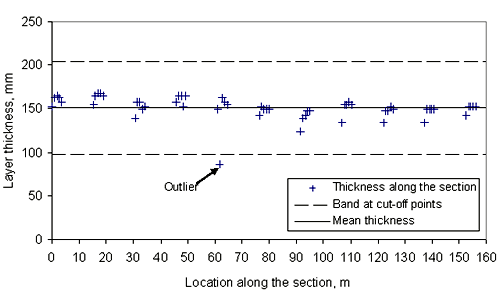Figure 19: Chart. Example of the binder course thickness measurements along SPS-6 Section 40_0608 with an apparent outlier.

Methodology to Identify Outliers

Because outliers can have a strong influence on both the skewness and kurtosis calculated for a data sample, the presence of a few outliers in a sample from a normal distribution may cause the sample to fail a normality test. Therefore, it is important to determine whether the apparent non-normality might be due to the presence of outliers. A data point was considered an outlier and removed from the analysis if the following is true:

• The absolute difference between an individual layer thickness measurement and the mean layer thickness, standardized (divided by) by the standard deviation, is greater than the 99.995 percentile (0.001 percent level of significance, two-sided test) of the t-distribution with n-1 degrees of freedom (df), where n is the number of data points in the sample.

The criterion is shown in equation format in figure 20.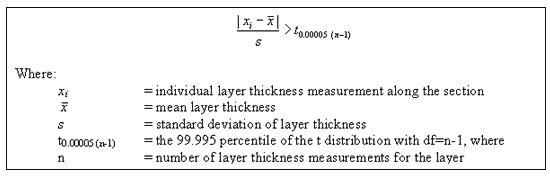Figure 20: Equation. Outlier definition criterion.

The t-values at the 99.995 percentile correspond to a level of significance of 0.01 percent for the two-sided t-test. The choice of a significance level of 0.01 percent is very conservative and was based on the fact that only "true" outliers (i.e., those that clearly do not belong in the same population with the other data points) should be excluded. If the distribution in reality is skewed, it is not desirable to cut out values based on a higher significance level, since the cut-off points are based on the (symmetric) normal distribution.

Note that the commonly used criterion (mean +/- 2 standard deviations) for identification of outliers was not used in this study. That criterion is based on a 5 percent significance level and the assumption that the distribution of the sample is normal. Because the standard deviation for LTPP sections is not known but estimated, the assumption of normality leads to the use of the t-distribution to create the 95 percent confidence interval. Based on the sample size, the t-distribution will provide a different number that the standard deviation is multiplied by to determine the cut-off points for outliers, as the examples in table 32 show.

Table 32. Multiplier for the standard deviation used in the outlier criterion based on t-distribution.
Sample SizeDegrees of FreedomMultiplier for the Standard Deviation
11 10 2.23
21 20 2.09
29 28 2.045
121 120 1.98
1.96

The following example using data from SPS-6 Section 40_0608 demonstrates the methodology and rationale used to determine the outlier points. The descriptive statistics for the binder course layer used in this example are provided in table 33. A scatter plot of all the thickness measurements is shown in figure 19.

Table 33. Descriptive statistics for the binder course layer, SPS-6 section 40_0608.
Section ID Layer Type Number of Layer Thickness Measurements Mean Layer Thickness, mm (in) Standard Deviation of Layer Thickness, mm (in)
40_0608 binder course 55 151 (5.951) 13 (0.501)

The data point identified as "Outlier" in figure 19 was evaluated to identify whether this point is a true outlier. The layer thickness value for this point is 86 mm (3.4 in), while the mean value for the sample is 151 mm (5.951 in). Using the criterion shown in figure 20, for the left side of the expression, we obtain the t-statistic value of 5.1. For the right side of the expression, the t-value of 4.2 was obtained at the 99.995 percentile of the t distribution with 54 (df = 55-1) degrees of freedom. Since the t-statistic of 5.1 is greater than the t-value of 4.2, this point was found to be an outlier using a cut-off point based on the t-distribution at a significance level of 0.01 percent with n-1degrees of freedom.

For the data in figure 19, the outlier point at 86 mm (3.4 in) could have been as large as 97 mm (3.8 in) and still would have been removed. In this particular data set, it may be desirable to remove points even greater than 97 mm (3.8 in) because the data otherwise do not appear skewed. However, in the data sets where some skewness is present, removal of the data points on the outskirts of the distribution could bias the reliability of the distribution evaluation results. The following example is used to demonstrate this concern.

Three different layer thickness frequency distributions are presented in figures 21, 22, and 23. The distribution in figure 21 shows an example of the clear outlier point on the left side of the distribution. Here the layer thickness value of the outlying point is <20 mm, while layer thicknesses for the rest of the points range from 82 to 142 mm. However, for the figures 22 and 23, the question whether the leftmost point is an outlier, cannot be answered with the same degree of certainty. The leftmost point in the distribution provided in figure 22 is a questionable outlier. Here the layer thickness value of the outlying point is about 75 percent of the average of the layer thickness values of the other points. The leftmost point in the distribution provided in figure 23 may be a legitimate point of a skewed distribution. Here the layer thickness value of the outlying point is about 80 percent of the average of the layer thickness values of the other points. However, even at the very conservative level chosen, the outlying point in figure 22 was identified as an outlier while the outlying point in figure 23 was not. This example illustrates why it was necessary to set the level for declaring a point an outlier very conservatively (in order to not bias the analysis of distribution type) in this study.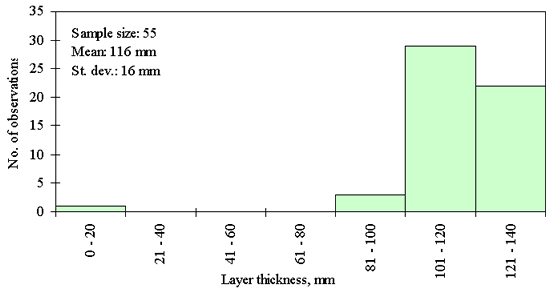Figure 21: Chart. Example of the AC surface and binder layer thickness distribution with clear outlier detection for the SPS-1 Section 30-0122.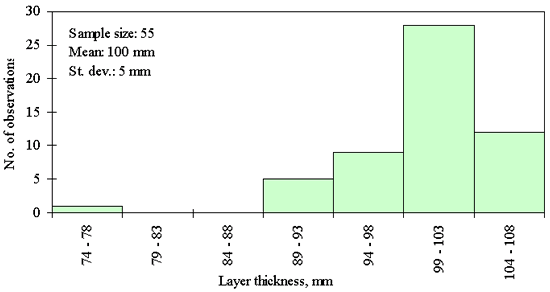Figure 22: Chart. Example of dense graded aggregate base layer thickness distribution with questionable outlier detection for the SPS-2 Section 20-0210.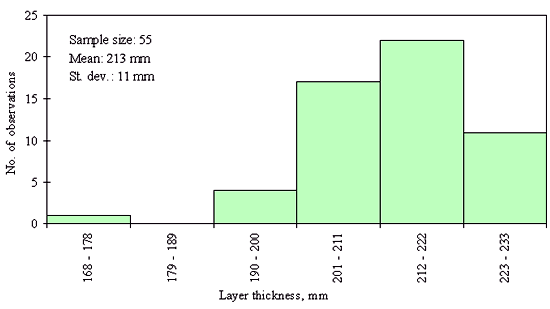Figure 23: Chart. Example of the dense graded aggregate base layer thickness distribution skewed to the left for the SPS-1 Section 20-0101.

This procedure for identification of the outliers was applied to each SPS structural layer with data available in the SPS*_LAYER_THICKNESS tables. In the whole data set of more than 55,000 data points, only 20 data points were excluded based on this criterion; the list of these excluded points is presented in table 34. These individual layer thicknesses were analyzed using special data distribution plots. The results show that these thickness values are likely to be errors in the database rather than actual thickness measurements. However, the review of the actual field data is required to confirm this conclusion. All anomalous or suspect data thickness values were reported back to the LTPP administrators for data review and possible correction of the thickness values in the LTPP layer thickness data tables.

Table 34. Identified outlier points.
Exp. Type STATE_CODE SHRP_ID Type Measured Thickness (outlier), mm Mean Thickness (with outliers), mm St. dev. (with outliers), mm Number of Measurements Standardized Difference t-value at 95.995 percent
SPS-6 400608BC8615113555.094.20
SPS-1 120102DGAB20830715556.694.20
SPS-1 300113DGAB1022102455 4.554.20
SPS-2 200210DGAB761005554.414.20
SPS-1 50122DGATB25 97 12 556.034.20
SPS-1 320105DGATB1501235555.034.20
SPS-1 350104DGATB19329725554.234.20
SPS-1 400116DGATB7130435556.634.20
SPS-2 50215PCCS32827512554.314.20
SPS-1 40116SB122956554.344.20
SPS-1 100103SB4612112556.144.20
SPS-1 300122SB1811616556.214.20
SPS-1 350105SB17011912554.244.20
SPS-1 390105SB4110111555.574.20
SPS-1 510116SB33 739554.224.20
SPS-8 29A802SB1421747634.234.16
SPS-8 390803SB18510115555.434.20
SPS-8 490803SB5810711554.294.20
SPS-6 29A606SC3611013555.714.20
SPS-6 290608SC1195912505.064.24

### Goodness-of-Fit between Experimental Data and Theoretical Statistical Distribution

Formulation of Statistical Hypothesis

Goodness-of-fit tests are used to evaluate how close the experimental data follow the assumed theoretical distribution. If the targeted theoretical distribution is a "normal" distribution, then the goodness-of-fit test becomes the test for normality. Such a test evaluates the closeness of the experimental data distribution to the normal distribution.

In the goodness-of-fit test, the null and alternative hypotheses are established first:

• The null hypothesis: "Measured field data follows a selected theoretical distribution, Φ."
• The alternative hypothesis: "Measured field data does not follow the theoretical distribution, Φ."

There are two kinds of errors that can be made in testing the hypothesis:

• Type I error: A true null hypothesis can be incorrectly rejected.
• Type II error: A false null hypothesis can fail to be rejected.

In the test of a hypothesis, it is desirable to have a small type I error and large power. Power is equal to 1 minus probability of a type II error and is defined as the probability of rejecting the null hypothesis when the alternative hypothesis is true. Testing whether a measured variable follows a certain theoretical distribution is not straightforward in the sense that the various tests are only powerful against certain types of alternative distributions.

Selection of the Targeted Theoretical Distribution

Based on the assumption that thickness measurements follow the same kind of distribution for any layer, one type of distribution was looked for. To determine the likely distribution shape, the measures of skewness and kurtosis were evaluated. The skewness of all samples ranged from -2.45 to +3.92 with a median of 0.024, while the kurtosis of all samples ranged from -1.56 to +17.78 with a median of -0.033. These measures indicate no particular skewness to either side or either particular long or short tails. This observation was confirmed by inspection of the layer thickness frequency distributions of each sample. While most of the reviewed layer thickness distributions looked fairly normal, as shown in figure 24, some samples had distributions that were skewed to one side or the other side, or looked rather uniformly distributed. Examples of different distribution shapes observed for the LTPP layer thickness measurements are provided in figures 23 to 26. The normal distribution was therefore selected as the most likely theoretical distribution to describe variability in the layer thickness along the LTPP section. This hypothesis was then tested using a goodness-of-fit test.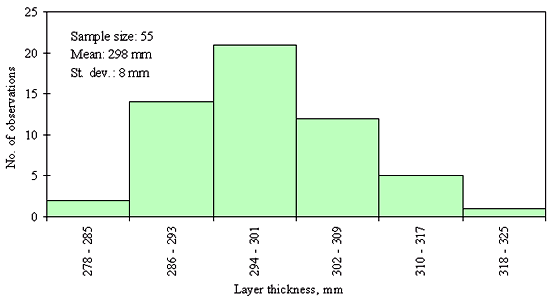Figure 24: Chart. Example of the normal layer thickness distribution for PCC surface layer, SPS-2, Section 10_0211.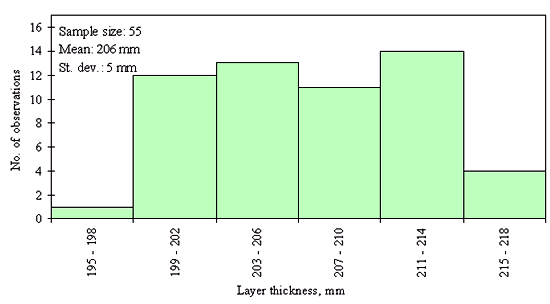Figure 25: Chart. Example of the uniform layer thickness distribution for dense graded aggregate base, SPS-1, Section 12_0101.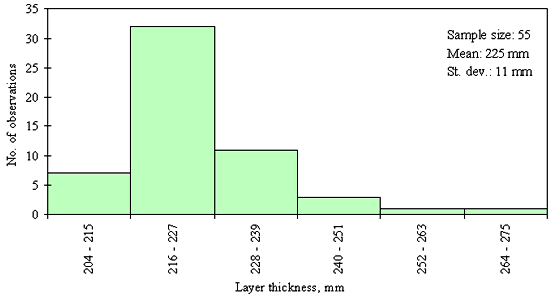Figure 26: Chart. Example of the layer thickness distribution skewed to the right for PCC surface layer, SPS-2, Section19_0213.

Selection of Testing Procedure

The goodness-of-fit test between assumed theoretical distribution and distribution of the observed data could be done using several methods including:

• Chi-square test
• Kolmogorov-Smirnov test

For the normal distribution more goodness-of-fit methods are available, including:

• Shapiro-Wilk's test
• Tests of kurtosis and skewness

To select the best applicable testing procedure, the LTPP layer thickness data characteristics were analyzed first. Based on the data review the following was established:

• Layer thickness values are measured at multiple locations along the LTPP section.
• Most of layer thickness distributions look fairly normal.
• There is a large number of same thickness measurements (many "ties") in a section.
• The number of data points and locations are different from one section to another and between different experiments.

The assumptions and requirements of different goodness-of-fit tests were reviewed from the point of their applicability and the robustness of the procedure when it is applied to the LTPP layer thickness data. The goal of this review was to find a procedure that could be uniformly used for all the sections with variable number of data points without compromising the test accuracy and without violating any of the underlying test assumptions.

For most theoretical distributions, the choice is limited to tests like the Kolmogorov-Smirnov test or the chi-square goodness-of-fit test . The advantage of the Kolmogorov-Smirnov test is that unlike the chi-square test it does not have strict rules on the required number of data groups and minimum theoretical frequencies that have to be satisfied in order for the test to be meaningful. The Kolmogorov-Smirnov test could be done for the samples with as few as five observations. The Kolmogorov-Smirnov test is also more powerful than the chi-square test.

If the null hypothesis is that the measured variable follows a normal distribution, there are more powerful tests available, such as the Shapiro-Wilk's test , the test of skewness or the third sample moment test and the test of kurtosis or the fourth sample moment test . The latter two tests work for a sample with nine observations or more. These tests are preferred to the Kolmogorov-Smirnov test because of the increased power  they provide. For a test to work well, it should have high power against all possible alternatives, which is not true for either the Kolmogorov-Smirnov test or the chi-square goodness-of-fit test. For the LTPP layer thickness data the Shapiro-Wilk's test was not appropriate, due to the many thickness measurement values that were the same (many "ties" ) for a given pavement layer and LTPP section.

The following table 35 provides a summary of the pros and cons of the reviewed goodness-of-fit testing methods.

Table 35. Evaluation summary of the goodness-of-fit testing methods.
Evaluation Criteria Chi-Square test Kolmogorov-Smirnov test Sharpiro-Wilk test Skewness-and-Kurtosis test
Test power (for normality only)very poor poor high high
Minimum number of observations25 5 3 9
Minimum number of observations in a single bin5 no restriction no restriction no restriction
Handling of "ties"high high poor high

Based on the review of different goodness-of-fit tests' procedures and analysis of the available layer thickness data, the following conclusions were derived:

• Goodness-of-fit tests are generally only powerful against certain alternative distributions - that is the reason why so many tests have been developed.
• For testing distribution normality, no other tests are as well rounded as the Sharpiro-Wilk test or the Skewness and Kurtosis tests.
• The Sharpiro-Wilk test doesn't handle ties well - which leaves the Skewness and Kurtosis tests as the best alternative for evaluation of within-section layer distribution normality.

The combined skewness and kurtosis test was selected for the evaluation of layer thickness distribution normality. Rejection in either skewness or kurtosis test was considered as a rejection of normality altogether. For example, for a sample to be considered as normally distributed, the analysis of data should pass both the skewness and the kurtosis tests for a selected level of significance.

Selection of the Level of Significance

The level of significance of 1 percent was chosen for the goodness-of-fit tests. The following considerations were taken into account in selecting this desired level of significance:

• In the test of a hypothesis, it is desirable to have a small type I error and large power; however, that cannot happen simultaneously. A compromise is found by setting the level of significance (or type I error) to either 5 percent or 1 percent, or even less.
• In many cases a 5 percent level is reasonable. In these cases, when testing a null hypothesis the researchers very frequently put forward a null hypothesis in the hope that they can discredit it.
• In the case of the goodness-of-fit test, the null hypothesis is that the distribution of the field data and the theoretical normal distribution are the same and the desire is not to reject (or fail-to-reject) this hypothesis.
• A rejection of a null hypothesis is a much stronger statement than a fail-to-reject outcome. A rejection of a null hypothesis says we are certain (at the specified significance level) that the null hypothesis is not true.
• A failure-to-reject means either there was not enough evidence to indicate the discrepancy or the discrepancy was really not there.
• In lieu of the problems with power of the goodness-of-fit tests, it is better to be slightly conservative and use a 1 percent significance level. The lower the significance level, the more the data must diverge from the null hypothesis to be significant.
• For the goodness-of-fit test, in case of rejection, we are 99 percent certain that the distribution is not normal.

Procedures for the Skewness and Kurtosis Test

Based on the assessment of the LTPP layer thickness data from the SPS*_LAYER_THICKNESS tables, a procedure based on the combination of skewness and kurtosis tests was selected as the most appropriate for ascertaining whether the frequency distributions of layer thickness measurements taken along the LTPP section follow a normal distribution. In this procedure, for a sample not to be rejected (as normally distributed), the layer thickness measurements sample should pass both the skewness and the kurtosis tests for a selected level of significance of 1 percent.

The procedure used for the combined skewness and kurtosis test is outlined in the flowchart in figure 27. Detailed statistical formula used to compute test parameters are provided in Appendix B.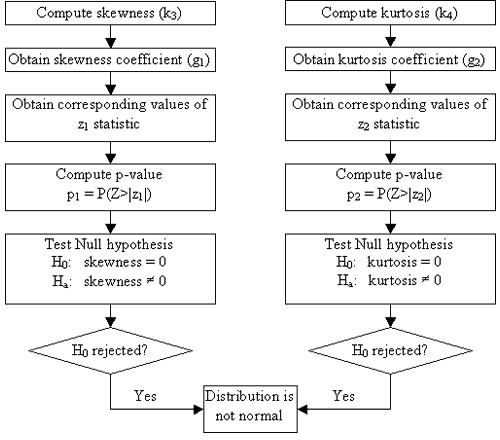Figure 27: Chart. Flowchart of the kurtosis and skewness test procedures used for the test of layer thickness distribution normality.

The skewness and kurtosis tests are based on evaluations of the third and fourth moments of the measurement distribution. The distribution is not rejected for being normally distributed if the absolute values of the z1- and z2-statistics computed separately based on skewness and kurtosis values are less than the Z-value of 2.57.

Z-value is obtained from the standard normal distribution, assuming a 1 percent level of significance. If a sample follows the standard normal distribution, the value Z=2.57 describes the distribution with 0.5 percent of the all the values from the sample greater than 2.57 and 0.5 percent of the values smaller than -2.57. Thus, when Z is equal to 2.57 the level of significance is 1 percent.

The z1- and z2-statistics are used to obtain the p-values (the probability that values of the standard normal distribution are more extreme than the computed z1- and z2-statistics). The p-values are defined in figure 28, as follows.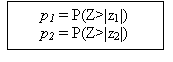Figure 28: Equation. Definition of p-values.

Based on the selected 1 percent level of significance, if p1- and p2-values are larger than 1 percent or equivalently if |z1| and |z2| < 2.57, we fail to reject that the data follow a normal distribution.

Example of the Kurtosis and Skewness Tests

The following example provides the comparison of the kurtosis and skewness test results obtained for the same binder course layer in the SPS-6 Section 40_0608, including and excluding an obvious outlier thickness measurement (86-mm [3.4-in] outlier thickness for a sample with 151-mm [5.951-in] mean thickness). Table 36 provides the summary of the test results.

Table 36. Kurtosis and skewness test results summary for binder course layer, SPS-6 Section 40_0608.
Sample Characteristic Sample Size, n Mean Sample Thickness,mm Standard Deviation, mm g1 g2 z1 z2 Z-value Is Normal?
Outlier included in the analysis55 151.15 12.72 -2.60 11.74 -5.51 4.80 2.57 No
Outlier excluded from the analysis54 152.35 9.17 -0.57 0.68 -1.77 1.14 2.57 Yes

When the outlier point was excluded, the mean does not change much while the standard deviation becomes 0.7 times smaller, and the skewness (g1) and the kurtosis (g2) change considerably. For this example, the exclusion of the outlying data point means that the tests for normality change from reject to not reject.

Results of the Kurtosis and Skewness Test of Normality for SPS Structural Layers

Kurtosis and skewness tests of normality were used to evaluate whether the experimental layer thickness data follow the theoretical normal distribution. A total of 1,047 layer thickness samples from the SPS experiments were considered for the analysis. Based on the number of available observations per sample, 13 samples were excluded from the analysis. These samples had fewer than 9 observations-the minimum number required for the kurtosis and skewness tests. All the samples were tested assuming the same evaluation criterion at 1 percent level of significance. The procedure for the kurtosis and skewness tests of normality described in the previous section was utilized.

The results of the kurtosis and skewness tests for different pavement material and layer types indicate that, based on the selected 1 percent level of significance, overall 84 percent of all layer thickness frequency distributions were not rejected for being normally distributed. This finding indicates that in general it is reasonable to assume that the layer thickness measurements taken along the section are normally distributed, but in a small number of sections this is not so. The distribution normality evaluations are summarized in table 37 by SPS experiment number and by layer and material type, respectively.

Table 37. Summary of the normality evaluation results.
Experiment Number of Layers Not Rejected (Normal) Rejected (Not Normal)
AC_SURFACE_COURSE
SPS-59378 (83.9 %)15 (16.1 %)
SPS-63630 (83.3 %)6 (16.7 %)
SURFACE_AND_BINDER
SPS-1167136 (81.4 %)31 (18.6 %)
SPS-82220 (90.9 %)2 (9.1 %)
PERM_ASPH_TREAT_BASE
SPS-18372 (86.8 %)11 (13.2 %)
SPS-24641 (89.1 %)5 (10.9 %)
PCC_SURFACE
SPS-2139102 (73.4 %)37 (26.6 %)
SPS-72423 (95.8 %)1 (4.2 %)
SPS-81412 (85.7 %)2 (14.3 %)
LEAN_CONCRETE
SPS-24840 (83.3 %)8 (16.7 %)
DENSE_GRD_ASPH_TREAT_BASE
SPS-19787 (89.7 %)10 (10.3 %)
SPS-19784 (86.6 %)13 (13.4 %)
SPS-28470 (83.3 %)14 (15.5 %)
SPS-83830 (79.0 %)8 (21.0 %)
BINDER_COURSE
SPS-53330 (87.9 %)3 (12.1 %)
SPS-61312 (92.3 %)1 (7.7 %)

Figures 29 through 44 provide examples of layer thickness frequency distributions obtained from the elevation measurements data for different layer and material types evaluated in the goodness-of-fit study. The data used to create these frequency distributions were determined to be reasonably normal based on skewness and kurtosis tests at selected level of significance. Theoretical normal distributions are superimposed over field frequency data to provide means for visual comparison between field data and theoretical distributions.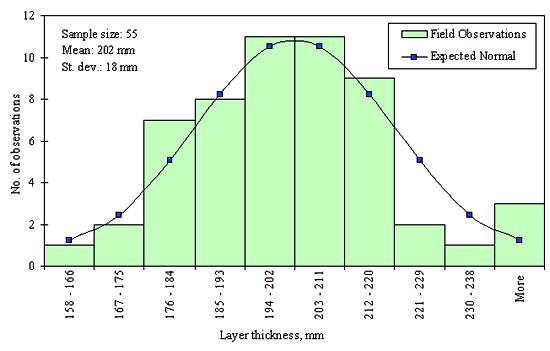Figure 29: Chart Example distribution of layer thickness measurements along the section for the DGAB layer for the SPS-1 Section 35-0108.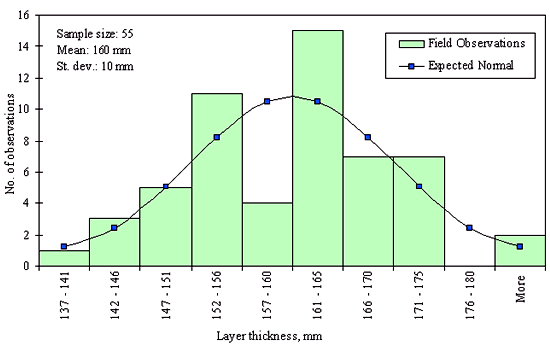Figure 30: Chart. Example distribution of layer thickness measurements along the section for the DGAB layer for the SPS-2 Section 19-0214.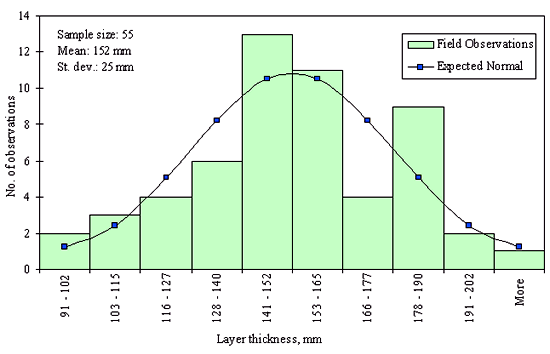Figure 31: Chart. Example distribution of layer thickness measurements along the section for the DGAB layer for the SPS-8 Section 08-0811.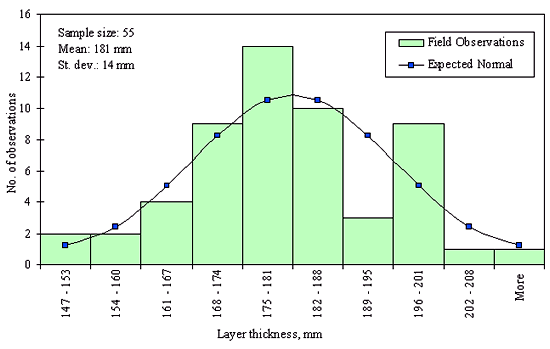Figure 32: Chart. Example distribution of layer thickness measurements along the section for the DGATB layer for the SPS-1 Section 22-0118.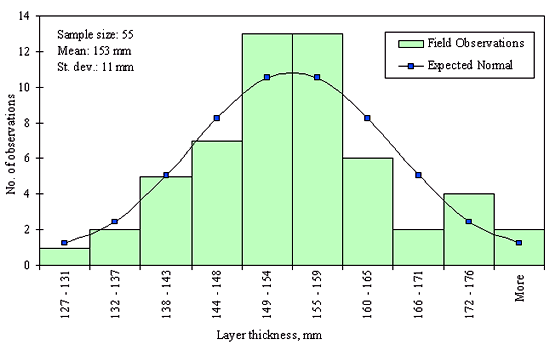Figure 33: Chart. Example distribution of layer thickness measurements along the section for the LC base layer for the SPS-2 Section 53-0207.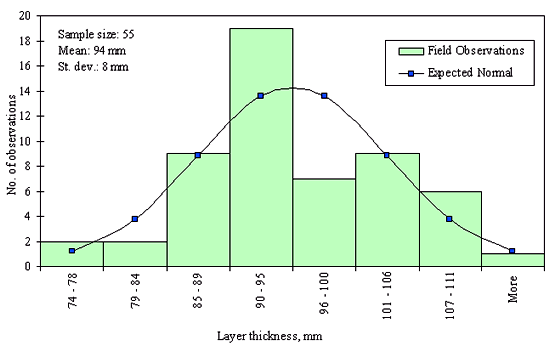Figure 34: Chart. Example distribution of layer thickness measurements along the section for the PATB layer for the SPS-1 Section 20-0112.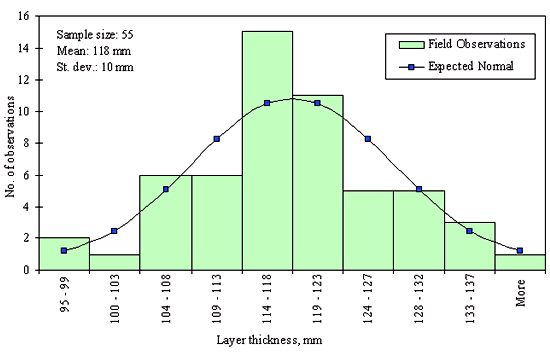Figure 35: Chart. Example distribution of layer thickness measurements along the section for the PATB layer for the SPS-2 Section 08-0224.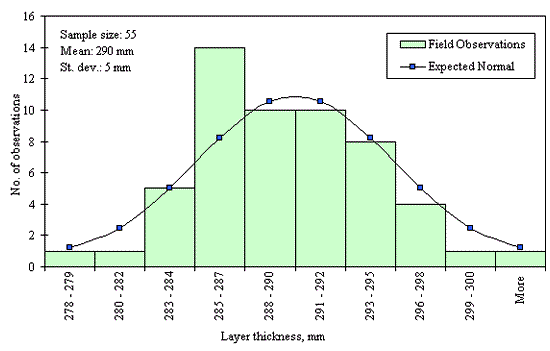Figure 36: Chart. Example distribution of layer thickness measurements along the section for the PCC surface layer for the SPS-2 Section 08-0215.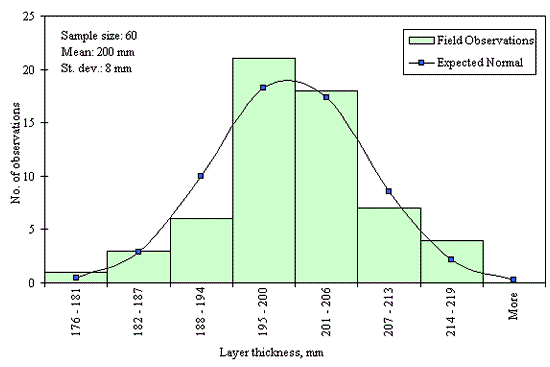Figure 37: Chart. Example distribution of layer thickness measurements along the section for the PCC surface layer for the SPS-8 Section 39-0809.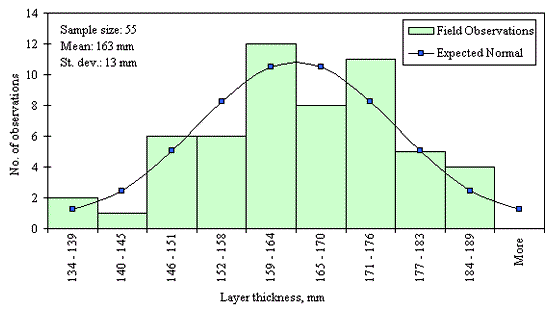Figure 38: Chart. Example distribution of layer thickness measurements along the section for the PCC surface layer for the SPS-7 Section 19-0706.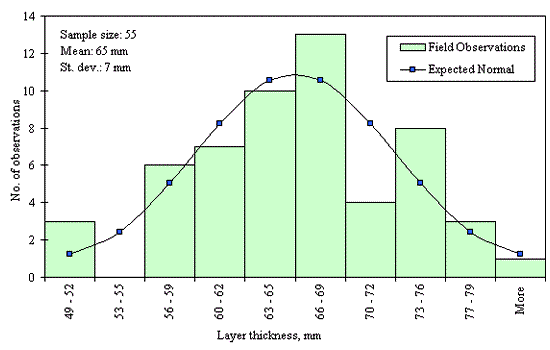Figure 39: Chart. Example distribution of layer thickness measurements along the section for the surface and binder layer for the SPS-1 Section 55-0118.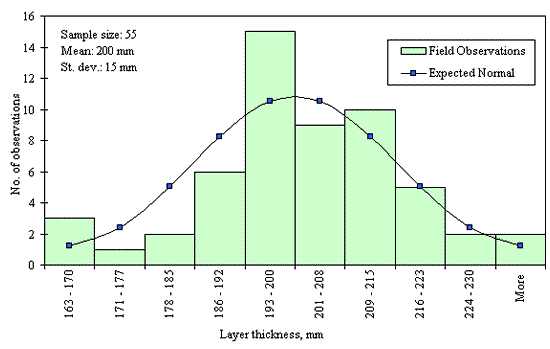Figure 40: Chart. Example distribution of layer thickness measurements along the section for the surface and binder layer for the SPS-8 Section 48-0802.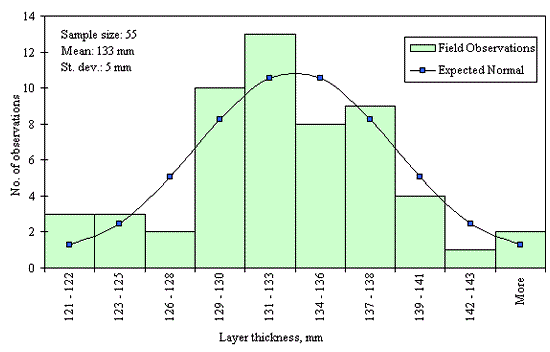Figure 41: Chart. Example distribution of layer thickness measurements along the section for the surface layer for the SPS-5 Section 35-0507.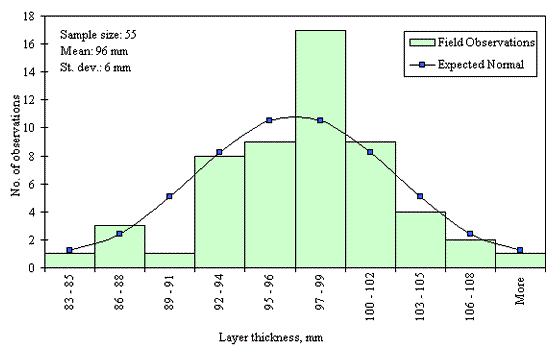Figure 42: Chart. Example distribution of layer thickness measurements along the section for the surface layer for the SPS-6 Section 42-0603.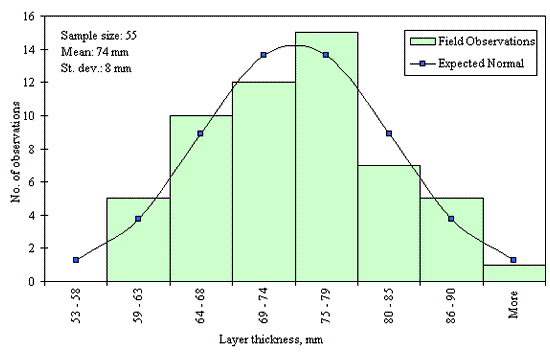Figure 43: Chart. Example distribution of layer thickness measurements along the section for the AC binder course for the SPS-5 Section 24-0504.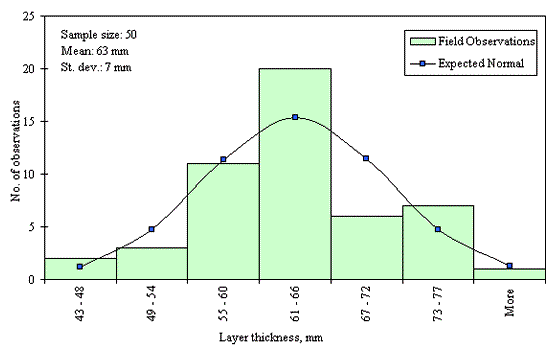Figure 44: Chart. Example distribution of layer thickness measurements along the section for the binder course for the SPS-6 Section 29-0607.

In addition to the kurtosis and skewness tests, Kolmogorov-Smirnov goodness-of-fit tests were carried out for the layer with thickness data in the SPS*_LAYER_THICKNESS tables. As was discussed earlier in the chapter, this testing procedure is not as powerful for testing normality as the kurtosis and skewness tests. A summary of the Kolmogorov-Smirnov goodness-of-fit testing procedure and evaluation results are presented in the Appendix C.

### Summary

In this chapter, layer thickness data from the SPS elevation measurements were analyzed to determine the extent to which the variation of layer thickness within a section follows typical statistical distributions. Data from the SPS*_LAYER_THICKNESS tables were obtained and reviewed. The layers used in the analysis include different material types and functional classifications, such as AC surface courses, combined AC surface and binder courses, AC binder courses, DGAB's, ATB's, LC bases, PCC surface layers, and PCC overlay layers. A methodology for identifying anomalous outlier points based on t-distribution was developed and utilized in evaluation of layer thickness data for each layer in the SPS*_LAYER_THICKNESS tables. All identified anomalous outlier data points were analyzed and reported to FHWA.

To assess layer thickness distribution characteristics, descriptive statistics such as mean, standard deviation, skewness, and kurtosis were computed for each section. Using descriptive statistics, the analysis of likely shapes of layer thickness distribution was conducted. The results of exploratory analysis indicated that, for most of the sections, the distribution is likely to be normal. To perform a more rigorous test of distribution normality, available procedures for goodness-of-fit tests were reviewed and their applicability to the evaluation of layer thickness data was evaluated. Based on the literature review, a combined test for skewness and kurtosis was selected to test normality of layer thickness distribution. A summary of the testing procedure was documented in this chapter. The analysis results for 1,034 SPS layers indicated that for 84 percent of all layer, frequency distributions of thickness values were not rejected for being normally distributed. Thus, LTPP data indicate that layer thickness variation within a section follows a normal distribution in most cases. These results would serve as a very important input to pavement engineering applications involving design reliability implementation.